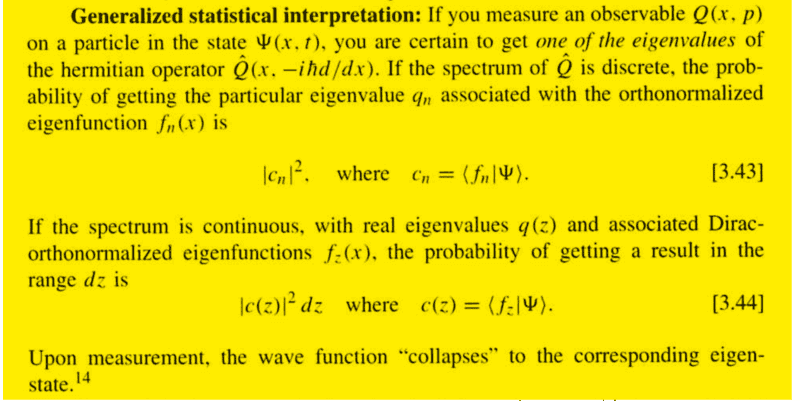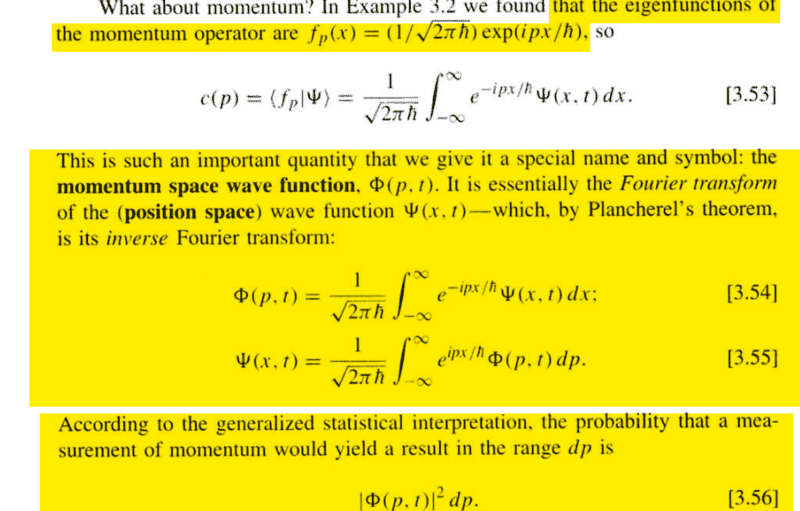# Momentum measurement of a particle in Quantum Mechanics

Pushoam

## Homework Statement

What will momentum measurement of a particle whose wave - function is given by ## \psi = e^{i3x} + 2e^{ix} ## yield?
Sketch the probability distribution of finding the particle between x = 0 to x = 2π.

## Homework Equations## The Attempt at a Solution

The eigenfunctions of momentum operator is given by ## A e^{ikx}## where k = ## \frac p {\hbar} ## with eigen value p = ## {\hbar} k##.
Thus eigenvalue of ##e^{i3x}## is ## 3 \hbar ## and ##e^{ix}## is ## \hbar ##. I feel myself tempted to take the eigenvalues of momentum operator to be discrete and say that the momentum measurement will be either ## 3 \hbar ## or ## \hbar ##.
As the eigenvalue of momentum operator is continuous, I should use equation. (3.56) to answer the question.

Assuming that the question asks to calculate the probability distribution at t = 0, probability density would be given by ## | \psi |^2 = 3 + 2 ( e^{ i2x} +e^{-i2x} )##., a complex function. But, the probability density should be a real valued function.
Is this correct?

#### Attachments

Last edited:

Staff Emeritus
Homework Helper
Gold Member
2021 Award
Plane waves are not normalizable so you really cannot write the probability in that manner (the wave function in momentum space is a sum of two delta functions). However, given the coefficients you should be able to deduce the probabilities (the coefficients are the probability amplitudes) by assuming that the total probability is one.

Pushoam
the coefficients are the probability amplitudes
How does one get to know this in case of continuous eigenvalues?

Staff Emeritus
Homework Helper
Assuming that the question asks to calculate the probability distribution at t = 0, probability density would be given by ## | \psi |^2 = 3 + 2 ( e^{ i2x} +e^{-i2x} )##., a complex function. But, the probability density should be a real valued function.
Is this correct?
I think you made a slight error. Anyway, your expression for ##| \psi |^2## is real.

Staff Emeritus
Homework Helper
Gold Member
2021 Award
I think you made a slight error. Anyway, your expression for ##| \psi |^2## is real.
However, it does not answer the question since it is the momenta that are asked for, not the position.

Staff Emeritus
Homework Helper